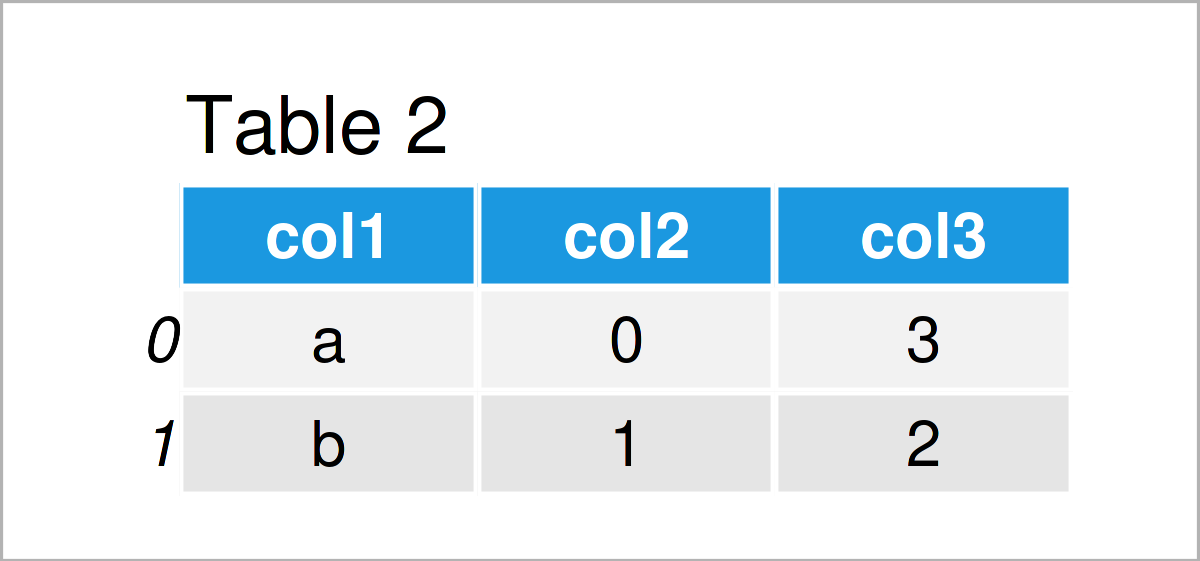# Combine pandas DataFrames with Same Column Names in Python (Example)

In this Python tutorial you’ll learn how to append two pandas DataFrames with the same columns.

The page is structured as follows:

Let’s get started!

## Exemplifying Data & Software Libraries

We first have to import the pandas library, to be able to use the functions that are included in the library.

`import pandas as pd                                      # Load pandas library`

In addition, consider the exemplifying two DataFrames below:

```data1 = pd.DataFrame({"col1":["y", "x", "y", "x", "y"],  # Create first pandas DataFrame
"col2":range(10, 15),
"col3":range(5, 0, - 1)})
print(data1)                                             # Print first pandas DataFrame``````data2 = pd.DataFrame({"col1":["a", "b"],                 # Create second pandas DataFrame
"col2":range(0, 2),
"col3":range(3, 1, - 1)})
print(data2)                                             # Print second pandas DataFrame```Tables 1 and 2 show that the example DataFrames consist of the three columns col1, col2, and col3 (i.e. they both have the same variable names). However, both of these data sets contain different values.

Let’s stack those two DataFrames on top of each other!

## Example: Combine Two pandas DataFrames with Same Column Names Using concat() Function

In this example, I’ll explain how to concatenate two pandas DataFrames with the same column names in Python.

To achieve this goal, we can use the concat function as illustrated below:

```data_concat = pd.concat([data1, data2],                  # Append two pandas DataFrames
ignore_index = True,
sort = False)
print(data_concat)                                       # Print combined DataFrame```The output of the previous Python programming code is shown in Table 3 – A vertically merged version of our two input data sets.

## Video & Further Resources

Have a look at the following video tutorial on my YouTube channel. In the video, I demonstrate the Python syntax of this tutorial.

In addition, you could have a look at the related tutorials on this homepage. I have released numerous articles already.

In this Python tutorial you have learned how to combine and join two pandas DataFrames with the same columns. In case you have further questions on how to create a union of two or more DataFrames, tell me about it in the comments section. Besides that, don’t forget to subscribe to my email newsletter in order to receive updates on the newest articles.

Subscribe to the Statistics Globe Newsletter